# Competitive On-line Prediction

In Competitive On-line Prediction one starts from a benchmark class of prediction strategies, and the goal is to design a prediction algorithm competitive with the best strategies in the benchmark class.

Consider a triple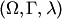, called a game of prediction. It consists of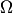, the set of possible outcomes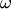,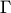, the set of possible predictions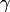, and the loss function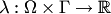. The loss functionmeasures the discrepancy between the current prediction and the actual outcome. The game is played in discrete time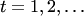. The cumulative loss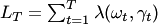is a measure of performance of the predictions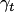on the realized outcomes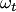. One can consider the performance of the best strategy in the chosen benchmark class and the performance of a prediction algorithm. Theoretical guarantees for the performance of the algorithm are often stated in the form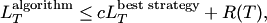or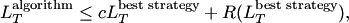where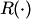is the regret term and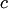does not depend on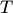. However, for "big" benchmark classes such regret terms are impossible, and the perfomance guarantee becomes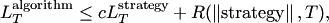which is required to hold for all strategies in the benchmark class; the regret term is now allowed to depend on the "complexity" (such as the norm) of the strategy.

Important subareas of competitive on-line prediction are:

The competitive on-line prediction can be applied, e.g., to:

There are many known techniques in competitive on-line prediction, such as:

Some techniques used in competitive on-line prediction are also standard in other areas of learning theory and statistics: